Subject: Linear Algebra Name: Hoda Who is asking: Student Level: All Question: I have a question about matrix multiplication; well, actually, matrix division. I am assuming that matrices are divided the same as they are multiplied; that is, row by column. But what happens if you have to divide by zero? How does this affect the resulting matrix? Specifically, the problem I am referring to: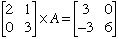To solve for A, you divide the right matrix by the one on the left. I come up with the following: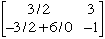What do I do with that 6/0? Hi Hoda, Unfortunately the matrix "division" operation you defined doesn't work the way you want it to. Compare your problem to a problem with numbers: 6x = 3 To solve for x=1/2 you could divide both sides by 6, or you could multiply both sides by (1/6). If you do the latter, you obtain (1/6)(6) x = (1/6)(3). Note that (1/6)(6)=1, and 1 times x is x. Hence we have x = (1/6)(3) = 1/2 The point of this very basic example is to illustrate the same technique for matrices. In matrix multiplication the identity matrix I palys the roll of 1 since, for any 2 by 2 matrix A, I x A = A. Note that if we multiply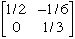by the matrix on the left side of the equation, we get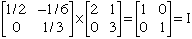the identity matrix I compare to (1/6) (6) = 1 Hence your system by pre-multiplying both sides by the above matrix. You get: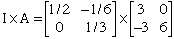which reduces to your answer,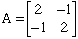For more information on such matrix inverses, and how to find them (it looks like we "pulled it out of a hat") consult a Linear Algebra text. Hope this gives you a start, Patrick and Penny Go to Math Central